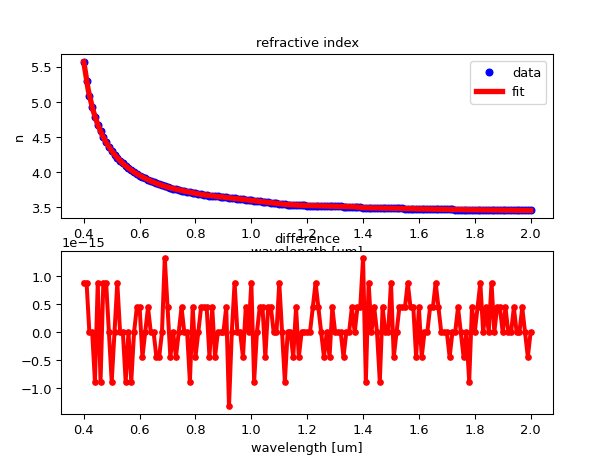# Material models¶

Material models for silicon (Si) and silicon dioxide (SiO2) are available in the SiFab PDK. These are based on textbook material data: the refractive indices n (real part) and k (imaginary part) for silicon and n for silicon dioxide.

## Model functions¶

The technology contains models as a function of wavelength in the form of functions:

• si_n(wavelength): silicon, real part of refractive index.
• si_k(wavelength): silicon, imaginary part of refractive index.
• sio2_n(wavelength): silicon dioxide, real part of refractive index.

For each of them, the wavelength should be specified in um.

## Data and fitting¶

The original data, as a function of wavelength, is saved in comma separated value (csv) files. These csv files are read and fitted when the PDK is loaded. The data is fitted using a cubic spline, resulting in a very small difference between the model and the data within the valid wavelength range:

# Plot silicon refractive index data and fitted model

from si_fab.technology.material_data import si_n_data, si_n
import pylab as plt
import numpy as np

wavs = np.linspace(si_n_data[0, 0], si_n_data[-1, 0], 100)
n = si_n(wavs)
n_at_data = si_n(si_n_data[:, 0])

plt.subplot(211)
plt.plot(si_n_data[:, 0], si_n_data[:, -1], 'bo', markersize=5, label='data')
plt.plot(wavs, n, 'r-', linewidth=4, label='fit')
plt.xlabel('wavelength [um]')
plt.ylabel('n')
plt.legend()
plt.title('refractive index')
plt.subplot(212)
plt.plot(si_n_data[:, 0], n_at_data - si_n_data[:, -1], 'ro-', markersize=4, linewidth=3)
plt.xlabel('wavelength [um]')
plt.title('difference')
plt.show()